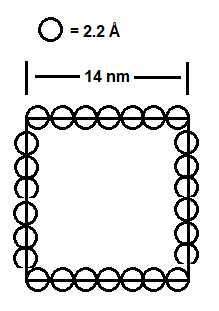# Problem: The diameter of a silicon atom is 2.22 Å. As of 2014, semiconductor chips as small as 14 nm { m nm}were commercially available. How many silicon atoms lie along the edge of one of these chips? Assume the semiconductor chip is a 2-dimensional square.

###### FREE Expert Solution

We have to calculate the number of silicon atoms that lie along the edge of a 14 nm silicon chip. Since the question asks us to assume that the chip is a two-dimensional square, we can consider the silicon atoms as circles. This could be roughly depicted by this diagram:We will solve this problem through these steps:

• Convert the diameter of a silicon atom to m units.
• Convert the length of the silicon chip to m units.
• Find the number of atoms that lie along the edge of the square.

98% (335 ratings)###### Problem Details

The diameter of a silicon atom is 2.22 Å. As of 2014, semiconductor chips as small as 14 nm were commercially available. How many silicon atoms lie along the edge of one of these chips? Assume the semiconductor chip is a 2-dimensional square.